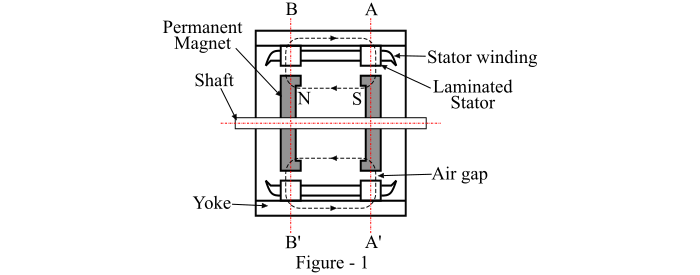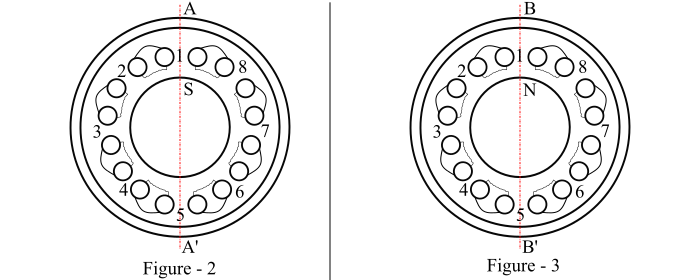# What is Hybrid Stepper Motor?

A hybrid stepper motor combines the features of the variable reluctance and the permanent magnet stepper motors. In the hybrid stepper motor, an axial permanent magnet is provided in the middle of the rotor. The permanent magnet is magnetised axially to produce a pair of poles marked N and S as shown in Figure-1.The end caps are fitted at both ends of the axial magnet. The end caps have equal number of teeth which are magnetised by the respective polarities of the axial magnet. The cross-sections of the two end caps of the rotor are shown in Figures-2 and 3.Let, the stator has 8-poles each with one coil and 5 teeth. Thus, there are 40-poles on the stator. Also, each end cap of the rotor has 50 teeth. Therefore, the step angle of the stepper motor is given by,

$$\mathrm{Step\:angle =\frac{𝑁_{𝑠} − 𝑁_{r}}{𝑁_{𝑠}𝑁_{𝑟}} \times 360°}$$

Where, NS is the number of stator poles and Nr is the number of rotor poles.

$$\mathrm{∴ Step\:angle =\frac{50 − 40}{50 \times 40}\times 360°= 1.8°}$$

The above figures show a hybrid stepper motor with a step angle of 1.8°. In Figure-2, it can be seen that the rotor teeth are in perfect alignment with stator teeth. However, from Figure-3, it can be seen that the rotor teeth are in alignment with stator slots. This can be described by stating that the teeth of the two end caps are displaced from each other by half a tooth pitch. The permanent magnet is axially magnetised, thus all the teeth on the left-end cap obtain south polarity, while all the teeth on the right-end cap obtain the north polarity.

The coils on the poles 1, 3, 5 and 7 are connected in series to form phase A. Similarly, the coils on the poles 2, 4, 6 and 8 are connected in series to form phase B. When the phase A is energised from a positive current, the stator poles 1 and 5 become south poles and the stator poles 3 and 7 become north poles. Thus, the rotor teeth with north polarity align with the stator poles 1 and 5, while the rotor teeth with south polarity align with the stator poles 3 and 7. Now, when the phase A is de-energised and the phase B is energised by a positive current, then the rotor will turn by a full step of 1.8° in the counter-clockwise direction.

Next, when the phase A is energised by a negative current, there is a further movement of the rotor by an angle of 1.8° in the same counter-clockwise direction. In order to turn the rotor further by 1.8°, the phase B is to be energised by the negative current. Hence, for producing the anticlockwise rotation of the rotor in steps of 1.8°, the phases are to be excited in the phase sequence +A, +B, -A, -B, +B, +A,…. Also, for producing the clockwise rotation of the rotor, the phase sequence should be +A, -B, +B, +A….

Now, if the excitation is removed from the hybrid stepper motor, the rotor continues to remain locked into the same position as before removal of the excitation. This is because the rotor is prevented to move in either direction by the detent torque or restraining torque produced by the permanent magnet.

## Advantages of Hybrid Stepper Motor

The advantages of hybrid stepper motors are given as follows −

• Hybrid stepper motors have small step length as compared to the variable reluctance stepper motors.

• Hybrid stepper motors have greater torque per unit volume than a VR stepper motor.

• Hybrid stepper motors provide dental torque with windings de-energised.

• Hybrid stepper motors have less tendency to resonate.

• Hybrid stepper motors have high efficiency at lower speeds and lower stepping rates.

## Disadvantages of Hybrid Stepper Motor

The hybrid stepper motor suffers from the following disadvantages −

• The performance of a hybrid stepper motor is affected by the change in magnetic strength.

• Hybrid stepper motor has high inertia and weight due to presence of rotor magnet.

• Hybrid stepper motors are more expensive than the variable reluctance stepper motor.